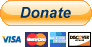2015-02-02T18:50:04Z
Originally Posted by: mauisys

Hi tha_Watcha,

I have the same results when changing the BaseURLMask setting and restarting the app.

When I add the web.config files to my site root all of my other hyperlinks no longer work. For example, when I click 'MUSIC', I just stay on the Home page.

Thanks,
Dave

Not ignoring that problem, but does the login then work for Facebook?•  mauisys
• YAF Camper Topic Starter
2015-02-02T18:52:48Z
Good question. I should have tried that...

I'll move some stuff around again and test.

Thanks.2015-02-02T18:54:36Z
Originally Posted by: mauisys

Good question. I should have tried that...

I'll move some stuff around again and test.

Thanks.

The reason i ask is because I had my stuff working for YAF too, but the URL rewriter ( and extension remover) caused my ASP.NET Content site navbar links to go bogus on me.•  mauisys
• YAF Camper Topic Starter
2015-02-03T03:33:07Z
Zero2Cool

Thanks a ton for your help!

I tried a bunch different configs and file arrangements with no luck.

At this point I am just going to stick with the Forum without the Facebook/Google/Twitter login feature - even though it's really cool.

Maybe at some point I'll try again.

Go Pack Go!2015-02-03T04:53:24Z•  mauisys
• YAF Camper Topic Starter
2015-02-03T20:24:03Z
I'll give it a try.

Is there installation/upgrade instructions for version 2.2.0?

Thanks.•  mauisys
• YAF Camper Topic Starter
2015-02-03T23:24:01Z
I installed version 2.2.0 from scratch.

Facebook login is almost working except when I try to login from Facebook I get a warning that the site is trying to collect the user birthdate. Is that a setting on the YAF or my Facebook app? I would like to shut that off so I don't have to submit my Facebook app for review.

Thanks.•  mauisys
• YAF Camper Topic Starter
2015-02-04T05:35:17Z
I am getting another error now after the re-install when I click on the Test Forum link.

Cannot use a leading .. to exit above the top directory.

This is the URL that is being called: http://www.signaldaddy.c...s/1-Test-Forum?name=Test  Forum

This is what my app.config looks like:
<appSettings>

</appSettings>

And URLRewite.config
<rewriter>
<!-- Standard URL Rewriting Format -->
<rewrite url="^~/(.+?)?yaf_info?\.aspx\?i=([0-9]+?)&amp;url\=(.+)\$" to="~/\$1Default.aspx?g=info&amp;i=\$2&amp;url=\$3" processing="stop" />
<rewrite url="^~/(.+)?yaf_topics([0-9]+)p([0-9]+)(_([\p{Ll}\p{Lu}\p{Lt}\p{Lo}\p{Nd}\p{Pc}-]+))?\.aspx(\?(.+))?\$" to="~/\$1Default.aspx?g=topics&amp;f=\$2&amp;p=\$3&amp;\$7" processing="stop" />
<rewrite url="^~/(.+)?yaf_topics([0-9]+)(_([\p{Ll}\p{Lu}\p{Lt}\p{Lo}\p{Nd}\p{Pc}-]+))?\.aspx(\?(.+))?\$" to="~/\$1Default.aspx?g=topics&amp;f=\$2&amp;\$6" processing="stop" />
<rewrite url="^~/(.+)?yaf_forum([0-9]+)(_([\p{Ll}\p{Lu}\p{Lt}\p{Lo}\p{Nd}\p{Pc}-]+))?\.aspx(\?(.+))?\$" to="~/\$1Default.aspx?g=forum&amp;c=\$2&amp;\$6" processing="stop" />
<rewrite url="^~/(.+)?yaf_postst([0-9]+)find([-a-z]+)(_([\p{Ll}\p{Lu}\p{Lt}\p{Lo}\p{Nd}\p{Pc}-]+))?\.aspx(\?(.+))?\$" to="~/\$1Default.aspx?g=posts&amp;t=\$2&amp;find=\$3&amp;\$7" processing="stop" />
<rewrite url="^~/(.+)?yaf_postst([0-9]+)p([0-9]+)(_([\p{Ll}\p{Lu}\p{Lt}\p{Lo}\p{Nd}\p{Pc}-]+))?\.aspx(\?(.+))?\$" to="~/\$1Default.aspx?g=posts&amp;t=\$2&amp;p=\$3&amp;\$7" processing="stop" />
<rewrite url="^~/(.+)?yaf_postst([0-9]+)(_([\p{Ll}\p{Lu}\p{Lt}\p{Lo}\p{Nd}\p{Pc}-]+))?\.aspx(\?(.+))?\$" to="~/\$1Default.aspx?g=posts&amp;t=\$2&amp;\$6" processing="stop" />
<rewrite url="^~/(.+)?yaf_postsm([0-9]+)find([-a-z]+)(_([\p{Ll}\p{Lu}\p{Lt}\p{Lo}\p{Nd}\p{Pc}-]+))?\.aspx(\?(.+))?\$" to="~/\$1Default.aspx?g=posts&amp;m=\$2&amp;find=\$3&amp;\$7" processing="stop" />
<rewrite url="^~/(.+)?yaf_postsm([0-9]+)(_([\p{Ll}\p{Lu}\p{Lt}\p{Lo}\p{Nd}\p{Pc}-]+))?\.aspx(\?(.+))?\$" to="~/\$1Default.aspx?g=posts&amp;m=\$2&amp;\$6" processing="stop" />
<rewrite url="^~/(.+)?yaf_profile([0-9]+)(_([\p{Ll}\p{Lu}\p{Lt}\p{Lo}\p{Nd}\p{Pc}-]+))?\.aspx(\?(.+))?\$" to="~/\$1Default.aspx?g=profile&amp;u=\$2&amp;\$6" processing="stop" />
<rewrite url="^~/(.+)?yaf_([-0-9a-z_]+?)\.aspx\?(.+)?\$" to="~/\$1Default.aspx?g=\$2&amp;\$3" processing="stop" />
<rewrite url="^~/(.+)?yaf_([-0-9a-z_]+?)\.aspx\$" to="~/\$1Default.aspx?g=\$2" processing="stop" />

<!-- Advanced URL Rewriting Format -->
<rewrite url="^~/(.+?)?info?\?i=([0-9]+?)&amp;url\=(.+)\$" to="~/\$1Default.aspx?g=info&amp;i=\$2&amp;url=\$3" processing="stop" />
<rewrite url="^~/(.+)?topics/([0-9]+)-(([\p{Ll}\p{Lu}\p{Lt}\p{Lo}\p{Nd}\p{Pc}-]+))?(/page([0-9]+))(\?(.+))?\$" to="~/\$1Default.aspx?g=topics&amp;f=\$2&amp;p=\$6&amp;\$7" processing="stop" />
<rewrite url="^~/(.+)?topics/([0-9]+)-(([\p{Ll}\p{Lu}\p{Lt}\p{Lo}\p{Nd}\p{Pc}-]+))?(\?(.+))?\$" to="~/\$1Default.aspx?g=topics&amp;f=\$2&amp;\$6" processing="stop" />
<rewrite url="^~/(.+)?category/([0-9]+)-(([\p{Ll}\p{Lu}\p{Lt}\p{Lo}\p{Nd}\p{Pc}-]+))?(\?(.+))?\$" to="~/\$1Default.aspx?g=forum&amp;c=\$2&amp;\$6" processing="stop" />
<rewrite url="^~/(.+)?posts/t([0-9]+)findlastpost-(([\p{Ll}\p{Lu}\p{Lt}\p{Lo}\p{Nd}\p{Pc}-]+))?(\?(.+))?\$" to="~/\$1Default.aspx?g=posts&amp;t=\$2&amp;find=lastpost&amp;\$7" processing="stop" />
<rewrite url="^~/(.+)?posts/t([0-9]+)-(([\p{Ll}\p{Lu}\p{Lt}\p{Lo}\p{Nd}\p{Pc}-]+))?(/page([0-9]+))(\?(.+))?\$" to="~/\$1Default.aspx?g=posts&amp;t=\$2&amp;p=\$6&amp;\$8" processing="stop" />
<rewrite url="^~/(.+)?posts/t([0-9]+)-(([\p{Ll}\p{Lu}\p{Lt}\p{Lo}\p{Nd}\p{Pc}-]+))?(\?(.+))?\$" to="~/\$1Default.aspx?g=posts&amp;t=\$2&amp;\$6" processing="stop" />
<rewrite url="^~/(.+)?posts/m([0-9]+)findlastpost-(([\p{Ll}\p{Lu}\p{Lt}\p{Lo}\p{Nd}\p{Pc}-]+))?(\?(.+))?\$" to="~/\$1Default.aspx?g=posts&amp;m=\$2&amp;find=lastpost&amp;\$7" processing="stop" />
<rewrite url="^~/(.+)?posts/m([0-9]+)-(([\p{Ll}\p{Lu}\p{Lt}\p{Lo}\p{Nd}\p{Pc}-]+))?(\?(.+))?\$" to="~/\$1Default.aspx?g=posts&amp;m=\$2&amp;\$6" processing="stop" />
<rewrite url="^~/(.+)?profile/([0-9]+)-(([\p{Ll}\p{Lu}\p{Lt}\p{Lo}\p{Nd}\p{Pc}-]+))?(\?(.+))?\$" to="~/\$1Default.aspx?g=profile&amp;u=\$2&amp;\$6" processing="stop" />
<rewrite url="^~/forum(.+)?\?(.+)?\$" to="~/forum/Default.aspx?g=\$1&amp;\$2" processing="stop" />
<rewrite url="^~/forum(.+)?\.aspx\$" to="~/forum/Default.aspx?g=\$1" processing="stop" />
<rewrite url="^~/forum(.+)?\$" to="~/forum/Default.aspx?g=\$1" processing="stop" />
</if>
</rewriter>